# 5.3: Polarity and Intermolecular Forces

•• Contributed by Allison Soult
• Senior Lecturer (Chemistry) at University of Kentucky

Learning Outcomes

• Define electronegativity.
• Describe how the electronegativity difference between two atoms in a covalent bond results in the formation of a nonpolar covalent, polar covalent, or ionic bond.
• Predict polarity of a molecule.
• Describe how molecular geometry plays a role in determining whether a molecule is polar or nonpolar.
• Distinguish between the following three types of intermolecular forces: dipole-dipole forces, London dispersion forces, and hydrogen bonds.
• Identify types of intermolecular forces in a molecule.
• Describe how chemical bonding and intermolecular forces influence the properties of various compounds.

In an ionic bond, one or more electrons are transferred from one atom to another. In a covalent bond, one or more pairs of electrons are shared between atoms. However, bonding between atoms of different elements is rarely purely ionic or purely covalent.

## Bond Polarity

Bond polarity is determined by the difference in electronegativity and is defined as the relative ability of an atom to attract electrons when present in a compound. The electronegativities of various elements are shown below. Note that elecronegativity values increase from left to right and from bottom to top on the periodic table. The degree to which a given bond is ionic or covalent is determined by calculating the difference in electronegativity between the two atoms involved in the bond.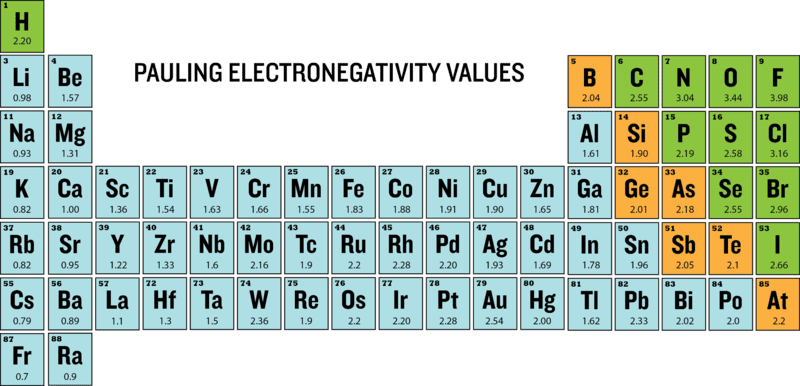Figure $$\PageIndex{1}$$: Electronegativities of the elements.

As an example, consider the bond that occurs between an atom of potassium and an atom of fluorine. Using the table, the difference in electronegativity is $$4.0 - 0.8 = 3.2$$. Because the difference in electronegativity is relatively large, the bond between the two atoms is primarily ionic. Since the fluorine atom has a much larger attraction for electrons than the potassium atom does, the valence electron from the potassium atom is considered to have completely transferred to the fluorine atom. The figure below shows how the difference in electronegativity relates to the ionic or covalent character of a chemical bond.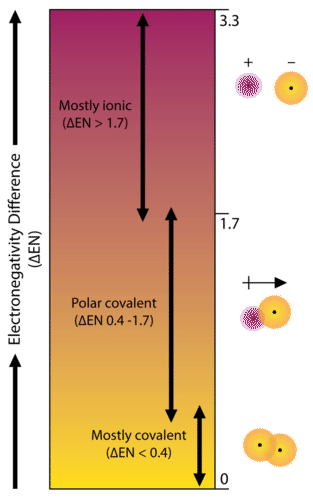Figure $$\PageIndex{2}$$: The difference in electronegativity between the two elements involved in a chemical bond is predictive of the type of bond made by those two atoms. A small difference (<0.4) results in a nonpolar covalent bond, an intermediate difference (0.4 - 1.7) results in a polar covalent bond, and a large difference (>1.7) results in an ionic bond.

According to the figure above, a difference in electronegativity ($$\Delta$$ EN) greater than 1.7 results in a bond that is mostly ionic in character.

### Nonpolar Covalent Bonds

A bond in which the electronegativity difference is less than 1.7 is considered to be mostly covalent in character. However, a distinction is often made between two general types of covalent bonds. A nonpolar covalent bond is a covalent ond in which the onding electrons are shared equally between the two atoms. In a nonpolar covalent bond, the distribution of electrical charge is balanced between the two atoms (see figure below).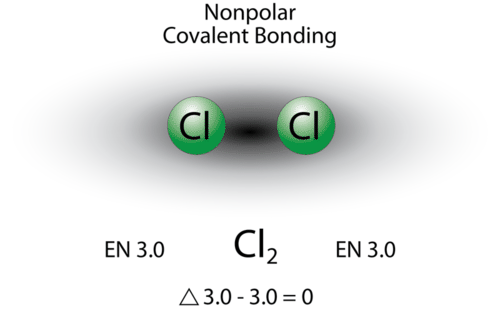Figure $$\PageIndex{3}$$: A nonpolar covalent bond is one in which the shared electrons are distributed equally between the two atoms.

The two chlorine atoms share the pair of electrons in the single covalent bond equally, and the electron density surrounding the $$\ce{Cl_2}$$ molecule is symmetrical. Any diatomic molecule in which the two atoms are the same element must be joined by a nonpolar covalent bond.

There are seven diatomic elements, which are elements whose natural form is of a diatomic molecule. They are hydrogen $$\left( \ce{H_2} \right)$$, nitrogen $$\left( \ce{N_2} \right)$$, oxygen $$\left( \ce{O_2} \right)$$, fluorine $$\left( \ce{F_2} \right)$$, chorine $$\left( \ce{Cl_2} \right)$$, bromine $$\left( \ce{Br_2} \right)$$, and iodine $$\left( \ce{I_2} \right)$$. By forming a diatomic molecule, both atoms in each of these molecules satisfy the octet rule, resulting in a structure that is much more stable than the isolated atoms

Notice from the figure above that molecules in which the electronegativity difference is very small (<0.4) are also considered nonpolar covalent. An example would be a bond between chlorine and bromine ($$\Delta$$ EN $$= 3.16 - 2.96 = 0.20$$).

### Polar Covalent Bonds

A bond in which the electronegativity difference between the atoms is between 0.4 and 1.7 is called a polar covalent bond. A polar covalent bond is a covalent bond in which the atoms have an unequal attraction for electrons, so the sharing is unequal. In a polar covalent bond, sometimes simply called a polar bond, the distribution of shared electrons within the molecule is no longer symmetrical (see figure below).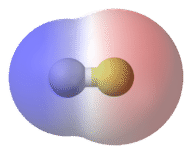Figure $$\PageIndex{4}$$: In the polar covalent bond of $$\ce{HF}$$, the electron density is unevenly distributed. There is a higher density (red) near the fluorine atom, and a lower density (blue) near the hydrogen atom.

The hydrogen fluoride molecule has an electronegativity difference of 1.9, which places it in the category of being slightly ionic. However, the hydrogen ion $$\left( \ce{H^+} \right)$$ is so very small that it is not capable of adopting the crystal lattice structure of an ionic compound. Hydrogen fluoride is a highly polar molecule. Because of its greater electronegativity, the electron density around the fluorine atom is much higher than the electron density around the hydrogen atom.

An easy way to illustrate the uneven electron distribution in a polar covalent bond is to use the Greek letter delta $$\left( \delta \right)$$ along with a positive or negative sign to indicate that an atom has a partial positive or negative charge.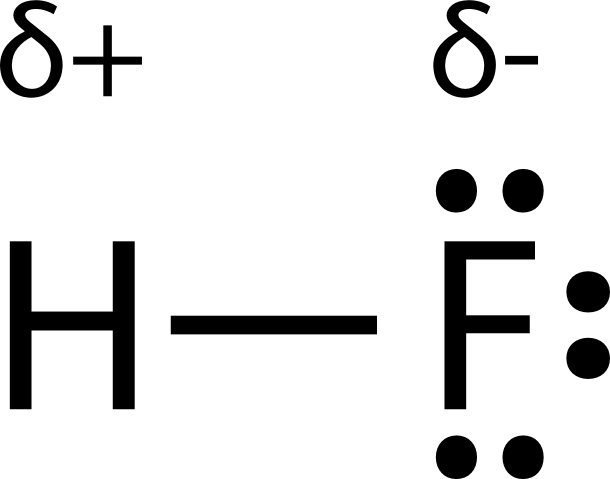The atom with the greater electronegativity acquires a partial negative charge, while the atom with the lesser electronegativity acquires a partial positive charge. The delta symbol is used to indicate that the quantity of charge is less than one. A crossed arrow can also be used to indicate the direction of greater electron density.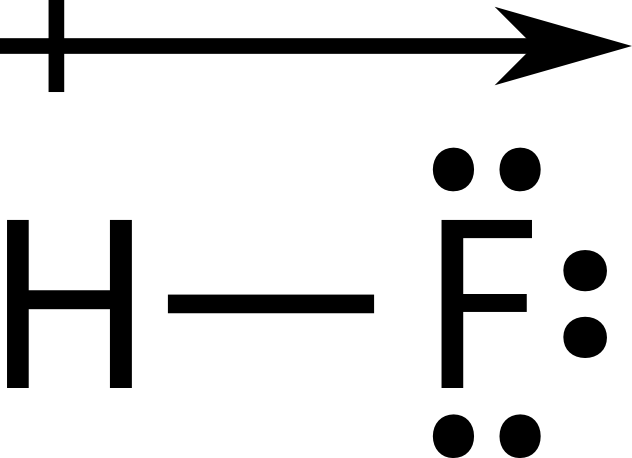Example $$\PageIndex{1}$$

Which type of bond will form between each of the following pairs of atoms?

1. $$\ce{C}$$ and $$\ce{O}$$
2. $$\ce{Na}$$ and $$\ce{N}$$
3. $$\ce{B}$$ and $$\ce{H}$$

Solution

Step 1: List the known quantities and plan the problem.

Known

Using the electronegativity chart:

• $$\ce{C} = 2.5, \: \ce{O} = 3.5$$
• $$\ce{Na} = 0.9, \: \ce{N} = 3.0$$
• $$\ce{B} = 2.0, \: \ce{H} = 2.1$$

Step 2: Solve.

Calculate the difference and use the diagram above to identify the bond type.

$3.5 - 2.5 = 1.0 \rightarrow \ce{C-O} \: \text{bond is polar covalent}$

$3.0 - 0.9 = 2.1 \rightarrow \ce{Na-N} \: \text{bond is ionic}$

$2.1 - 2.0 = 0.1 \rightarrow \ce{B-H} \: \text{bond is nonpolar covalent}$

Bonds between nonmetal atoms are generally covalent in nature (A and C), while bond between a metal atom and a nonmetal atom are generally ionic.

## Molecular Polarity

A polar molecule is a molecule in which one end of the molecule is slightly positive, while the other end is slightly negative. A diatomic molecule that consists of a polar covalent bond, such as $$\ce{HF}$$, is a polar molecule. The two electrically charged regions on either end of the molecule are called poles, similar to a magnet having a north and a south pole. A molecule with two poles is called a dipole. Hydrogen fluoride is a dipole. A simplified way to depict molecules is pictured below (see figure below).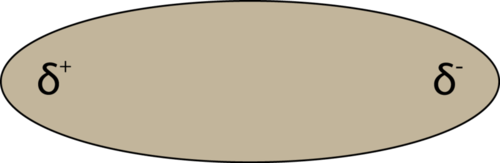Figure $$\PageIndex{5}$$: A molecular dipole results from the unequal distribution of electron density throughout a molecule.

When placed between oppositely charged plates, polar molecules orient themselves so that their positive ends are closer to the negative plate and their negative ends are closer to the positive plate (see figure below).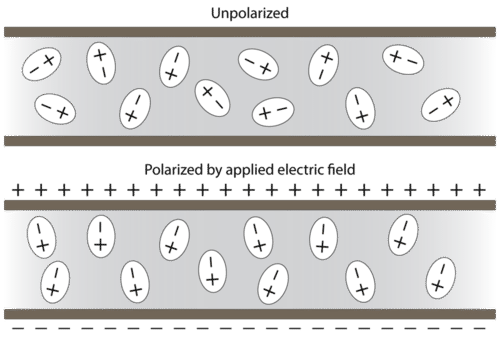Figure $$\PageIndex{6}$$: Polar molecules are randomly oriented in the absence of an applied electric field (top). In an electric field, the molecules orient themselves to maximize the attraction between opposite charges (bottom).

Experimental techniques involving electric fields can be used to determine if a certain substance is composed of polar molecules and to measure the degree of polarity.

For molecules with more than two atoms, the molecular geometry must also be taken into account when determining if the molecule is polar or nonpolar. Pictured below (see figure below) is a comparison between carbon dioxide and water. Carbon dioxide $$\left( \ce{CO_2} \right)$$ is a linear molecule. The oxygen atoms are more electronegative than the carbon atom, so there are two individual dipoles pointing outward from the $$\ce{C}$$ atom to each $$\ce{O}$$ atom. However, since the dipoles are of equal strength and are oriented in this way, they cancel each other out, and the overall molecular polarity of $$\ce{CO_2}$$ is zero.

Water is a bent molecule because of the two lone pairs on the central oxygen atom. The individual dipoles point from the $$\ce{H}$$ atoms toward the $$\ce{O}$$ atom. Because of the shape the dipoles do not cancel each other out, and the water molecule is polar. In the figure below, the net dipole is shown in blue and points upward.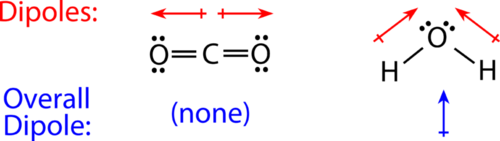Figure $$\PageIndex{7}$$: The molecular geometry of a molecule affects its polarity. In $$\ce{CO_2}$$, the two polar bonds cancel each other out, and the result is a nonpolar molecule. Water is polar because its bent shape means that the two polar bonds do not cancel.

Some other molecules are shown below (see figure below). Notice that a tetrahedral molecule such as $$\ce{CH_4}$$ is nonpolar. However, if one of the peripheral $$\ce{H}$$ atoms is replaced by another atom that has a different electronegativity, the molecule becomes polar. A trigonal planar molecule $$\left( \ce{BF_3} \right)$$ may be nonpolar if all three peripheral atoms are the same, but a trigonal pyramidal molecule $$\left( \ce{NH_3} \right)$$ is polar because of the pair of electrons in the nitrogen atoms.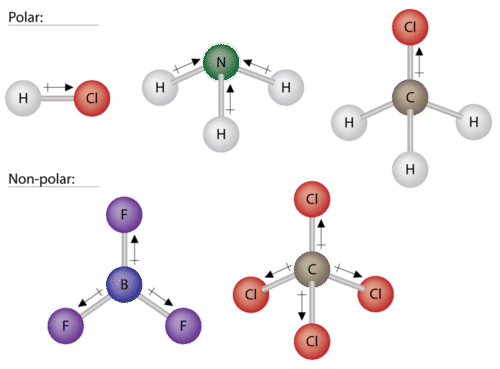Figure $$\PageIndex{8}$$: Some examples of polar and nonpolar molecules with various molecular geometries.

## Intermolecular Forces

Covalent and ionic bonds can be called intramolecular forces: forces that act within a molecule or crystal. Molecules also attract other molecules. Intermolecular forces are attractions that occur between molecules. Intermolecular forces are weaker than either ionic or covalent bonds. However, the varying strengths of different types of intermolecular forces are responsible for physical properties of molecular compounds such as melting and boiling points and the amount of energy needed for changes in state.

### London Dispersion Forces

Dispersion forces are the weakest of all intermolecular forces. They are often called London forces after Fritz London (1900 - 1954), who first proposed their existence in 1930. London dispersion forces are intermolecular forces that occur between all atoms and molecules due to the random motion of electrons.

For example, the electron cloud of a helium atom contains two electrons, and, when averaged over time, these electrons will distribute themselves evenly around the nucleus. However, at any given moment, the electron distribution may be uneven, resulting in an instantaneous dipole. This weak and temporary dipole can subsequently influence neighboring helium atoms through electrostatic attraction and repulsion. The formation of an induced dipole is illustrated below.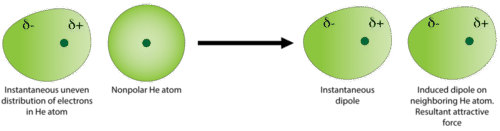Figure $$\PageIndex{9}$$: Random fluctuations in the electron density within the electron cloud of a helium atom results in a short-lived ("instantaneous") dipole. The attractive force between instantaneous dipoles and the resulting induced dipoles in neighboring molecules is called the London dispersion force.

The instantaneous and induced dipoles are weakly attracted to one another. The strength of dispersion forces increases as the total number of electrons in the atoms or nonpolar molecules increases. The halogen group consists of four elements that all take the form of nonpolar diatomic molecules. Listed below is a comparison of the melting and boiling points for each.

Table $$\PageIndex{1}$$: Melting and Boiling Points of Halogens
Molecule Total Number of Electrons Melting Point $$\left( ^\text{o} \text{C} \right)$$ Boiling Point $$\left( ^\text{o} \text{C} \right)$$ Physical State at Room Temperature
$$\ce{F_2}$$ 18 -220 -188 gas
$$\ce{Cl_2}$$ 34 -102 -34 gas
$$\ce{Br_2}$$ 70 -7 59 liquid
$$\ce{I_2}$$ 106 114 184 solid

The dispersion forces are strongest for iodine molecules because they have the greatest number of electrons. The relatively stronger forces result in melting and boiling points which are the highest of the halogen group. These forces are strong enough to hold iodine molecules close together in the solid state at room temperature. The dispersion forces are progressively weaker for bromine, chlorine, and fluorine, as illustrated by their steadily lower melting and boiling points. Bromine is a liquid at room temperature, while chlorine and fluorine are gases. Because gaseous molecules are so far apart from one another, intermolecular forces are nearly nonexistent in the gas state, and so the dispersion forces in chlorine and fluorine only become measurable as the temperature decreases and they condense into the liquid state.

### Dipole-Dipole Forces

Dipole-dipole forces are the attractive forces that occur between polar molecules (see figure below). A molecule of hydrogen chloride has a partially positive hydrogen atom and a partially negative chlorine atom. A collection of many hydrogen chloride molecules will align themselves so that the oppositely charged regions of neighboring molecules are near each other.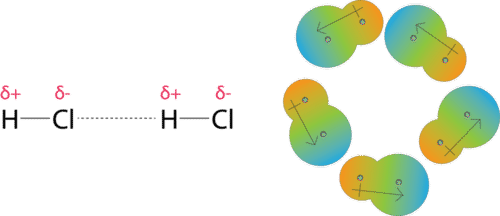Figure $$\PageIndex{10}$$: Dipole-dipole forces result from the attraction between the positive end of one dipole and the negative end of a neighboring dipole. Dipole-dipole forces are similar to ionic bonds, but because they involve only partial charges, they are much weaker.

### Hydrogen Bonding

The attractive force between water molecules is an unusually strong type of dipole-dipole interaction. Water contains hydrogen atoms that are bound to a highly electronegative oxygen atom, making for very polar bonds. The partially positive hydrogen atom of one molecule is then attracted to the oxygen atom of a nearby water molecule (see figure below).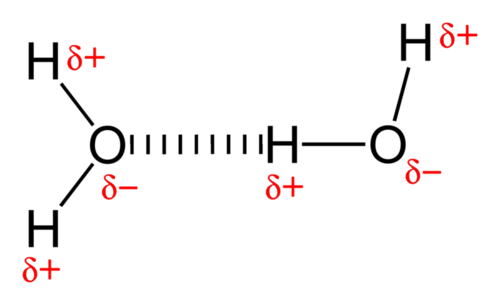Figure $$\PageIndex{11}$$: A hydrogen bond in water occurs between the hydrogen atom of one water molecule and the lone pair of electrons on the oxygen atom of a neighboring water molecule.

A hydrogen bond is an intermolecular attractive force in which a hydrogen atom, that is covalently bonded to a small, highly electronegative atom, is attracted to a lone pair of electrons on an atom in a neighboring molecule. Hydrogen bonds are very strong compared to other dipole-dipole interactions, but still much weaker than a covalent bond. A typical hydrogen bond is about $$5\%$$ as strong as a covalent bond.

Hydrogen bonding occurs only in molecules where hydrogen is covalently bonded to one of three elements: fluorine, oxygen, or nitrogen. These three elements are so electronegative that they withdraw the majority of the electron density from the covalent bond with hydrogen, leaving the $$\ce{H}$$ atom very electron-deficient. Because the hydrogen atom does not have any electrons other than the ones in the covalent bond, its positively charged nucleus is almost completely exposed, allowing strong attractions to other nearby lone pairs of electrons.

The hydrogen bonding that occurs in water leads to some unusual, but very important properties. Most molecular compounds that have a mass similar to water are gases at room temperature. However, because of the strong hydrogen bonds, water molecules are able to stay condensed in the liquid state. The figure below shows how its bent shape and the presence of two hydrogen atoms per molecule allows each water molecule to hydrogen bond with several other molecules.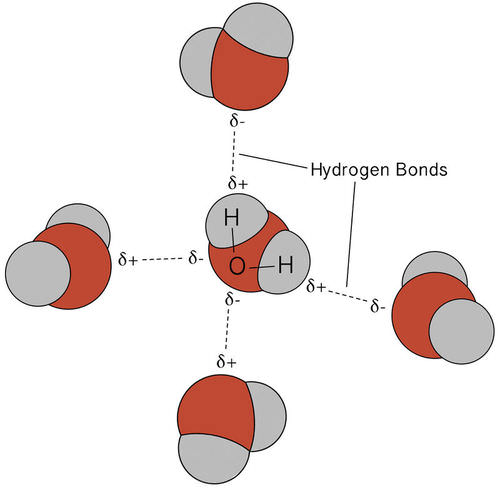Figure $$\PageIndex{12}$$: Multiple hydrogen bonds occur simultaneously in water because of its bent shape and the presence of two hydrogen atoms per molecule.

In the liquid state, the hydrogen bonds of water can break and reform as the molecules flow from one place to another. When water is cooled, the molecules begin to slow down. Eventually, when water is frozen to ice, the hydrogen bonds become more rigid and form a well-defined network (see figure below).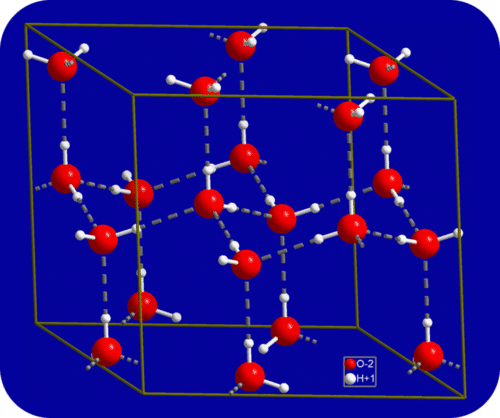Figure $$\PageIndex{13}$$: When water freezes to ice, the hydrogen bonding network becomes fixed until the ice melts. Each oxygen atom has an approximately tetrahedral geometry that includes two covalent bonds and two hydrogen bonds.

The bent shape of the molecules leads to gaps in the hydrogen bonding network of ice. Ice has the very unusual property that its solid state is less dense than its liquid state. As a result, ice floats in liquid water. Virtually all other substances are denser in the solid state than in the liquid state. Hydrogen bonds also play a very important biological role in the physical structures of proteins and nucleic acids.

## Boiling Points and Bonding Types

In order for a substance to enter the gas phase, its particles must completely overcome the intermolecular forces holding them together. Therefore, a comparison of boiling points is essentially equivalent to comparing the strengths of the attractive intermolecular forces exhibited by the individual molecules. For small molecular compounds, London dispersion forces are the weakest intermolecular forces. Dipole-dipole forces are somewhat stronger, and hydrogen bonding is a particularly strong form of dipole-dipole interaction. However, when the mass of a nonpolar molecule is sufficiently large, its dispersion forces can be stronger than the dipole-dipole forces in a lighter polar molecule. Thus, nonpolar $$\ce{Cl_2}$$ has a higher boiling point than polar $$\ce{HCl}$$.

Table $$\PageIndex{2}$$: Intermolecular Forces and Boiling Points
Substance Strongest Intermolecular Force Boiling Point $$\left( ^\text{o} \text{C} \right)$$
$$\ce{H_2}$$ dispersion -253
$$\ce{Ne}$$ dispersion -246
$$\ce{O_2}$$ dispersion -183
$$\ce{Cl_2}$$ dispersion -34
$$\ce{HCl}$$ dipole-dipole -85
$$\ce{HBr}$$ dipole-dipole -66
$$\ce{H_2S}$$ dipole-dipole -61
$$\ce{NH_3}$$ hydrogen bonding -33
$$\ce{HF}$$ hydrogen bonding 20
$$\ce{H_2O}$$ hydrogen bonding 100

## Supplemental Resources

• Electronegativity: www.chemguideco.uk/atoms/bond...elecroneg.html
• Intermolecular Bonding - van der Waals Forces: www.chemguidecouk/atoms/bonding/vdw.html
• Intermolecular Bonding - Hydrogen Bonds: www.chemguide.co.uk/bonding/hbond.html
• Ionic bond formation: www.dlt.ncssm/edu/core/Chapte...icBonding.html
• Polar covalent bond formation: http://www.dlt.ncssm.edu/core/Chapte...arBonding.html
• Nonpolar covalent bond formation: www.dlt.ncssm/edu/core/Chapte...ntBonding.html